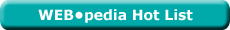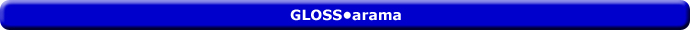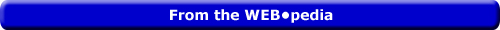Friday  March 31, 2023
 AmosWEB means Economics with a Touch of Whimsy!TOTAL REVENUE AND TOTAL COST: A profit-maximizing firm produces output where the difference between total revenue and total cost, that is economic profit, is the greatest. This total revenue and total cost approach to identifying profit-maximizing production can be accomplished using either a table of numbers of a set of curves. However, the end result is the same. Profit-maximizing production takes place at the quantity generating the greatest difference between total revenue and total cost. An added benefit of performing the analysis with curves, however, is the observation that profit-maximizing production occurs where the slopes of the total revenue and total cost curves are equal.Most Viewed (Number) Visit the WEB*pediaPROFIT CURVE: A curve that graphically represents the relation between economic profit earned by a firm and the quantity of output sold. This curve is constructed to capture the relation between profit and the level of output, holding other variables, especially those affecting the total revenue and total cost curves, constant. This is one of three methods typically used to determine the profit-maximizing quantity of output produced by a firm. The other two methods are total revenue and total cost and marginal revenue and marginal cost.Recommended Citation:

PROFIT CURVE, AmosWEB GLOSS*arama, http://www.AmosWEB.com, AmosWEB LLC, 2000-2023. [Accessed: March 31, 2023].

AmosWEB Encyclonomic WEB*pedia:

Additional information on this term can be found at:

WEB*pedia: profit curve

Search Again?PERFECT COMPETITION, PROFIT ANALYSIS

A perfectly competitive firm produces the profit-maximizing quantity of output that generates the highest level of profit. This profit approach is one of three methods that used to determine the profit-maximizing quantity of output. The other two methods involve a comparison of total revenue and total cost or a comparison of marginal revenue and marginal cost.BEIGE MUNDORTLE[What's This?] Today, you are likely to spend a great deal of time visiting every yard sale in a 30-mile radius trying to buy either a T-shirt commemorating yesterday or a pair of handcrafted oven mitts. Be on the lookout for crowded shopping malls.Your Complete ScopeA thousand years before metal coins were developed, clay tablet "checks" were used as money by the Babylonians."A leader, once convinced that a particular course of action is the right one, must . . . be undaunted when the going gets tough."-- President Ronald ReaganM&OManagement and OrganizationA PEDestrian's Guide Xtra CreditTell us what you think about AmosWEB. Like what you see? Have suggestions for improvements? Let us know. Click the User Feedback link.| | | | | | | | | | |
| | | |

Thanks for visiting AmosWEB